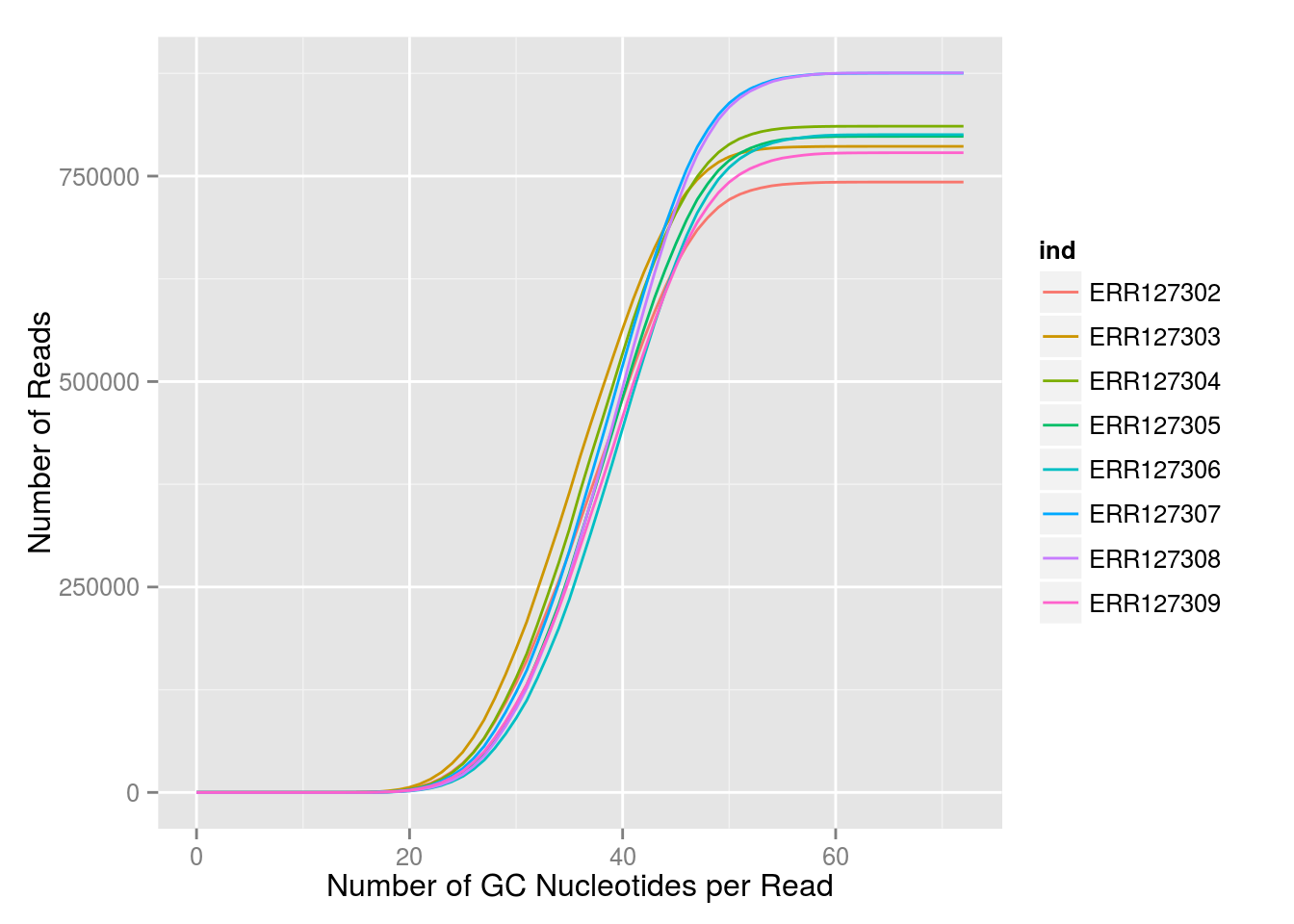Authors: Martin Morgan (mtmorgan@fhcrc.org), Sonali Arora (sarora@fredhutch.org)
Date: 19 June, 2015

The goal of this section is to learn to write correct, robust, simple and efficient R code. We do this through a couple of case studies.

## Priorities

1. Correct: consistent with hand-worked examples (identical(), all.equal())
2. Robust: supports realistic inputs, e.g., 0-length vectors, NA values, …
3. Simple: easy to understand next month; easy to describe what it does to a colleague; easy to spot logical errors; easy to enhance.
4. Fast, or at least reasonable given the speed of modern computers.

## Strategies

1. Profile
• Look at the script to understand in general terms what it is doing.
• Step through the code to see how it is executed, and to gain an understanding of the speed of each line.
• Time evaluation of select lines or simple chunks of code with system.time() or the microbenchmark package.
• Profile the code with a tool that indicates how much time is spent in each function call or line – the built-in Rprof() function, or packages such as lineprof or aprof
1. Vectorize – operate on vectors, rather than explicit loops

x <- 1:10
log(x)     ## NOT for (i in seq_along) x[i] <- log(x[i])
##   0.0000000 0.6931472 1.0986123 1.3862944 1.6094379 1.7917595 1.9459101
##   2.0794415 2.1972246 2.3025851
2. Pre-allocate memory, then fill in the result

result <- numeric(10)
result <- runif(1)
for (i in 2:length(result))
result[i] <- runif(1) * result[i - 1]
result
##   0.7386850875 0.3696589691 0.3542664926 0.0510763549 0.0254611460
##   0.0070750491 0.0038783618 0.0011608073 0.0007160663 0.0006206700
3. Hoist common sub-expressions outside of repeated calculations, so that the sub-expression is only calculated once
• Simple, e.g., ‘hoist’ constant multiplications from a for loop
• Higher-level, e.g., use lm.fit() rather than repeatedly fitting the same design matrix.
1. Re-use existing, tested code
• Efficient implementations of common operations – tabulate(), rowSums() and friends, %in%, …
• Efficient domain-specific implementations, e.g., snpStats for GWAS linear models; limma for microarray linear models; edgeR, DESeq2 for negative binomial GLMs relevant to RNASeq.
1. Re-think how to attack the problem
• Different implementations
• Alternative algorithms
1. Compile your script with the byte compiler
2. Use parallel evaluation
3. Speak in tongues – ‘foreign’ languages like C, Fortran

## Case study: from iteration to vectorization

Here’s an obviously inefficient function:

f0 <- function(n, a=2) {
## stopifnot(is.integer(n) && (length(n) == 1) &&
##           !is.na(n) && (n > 0))
result <- numeric()
for (i in seq_len(n))
result[[i]] <- a * log(i)
result
}

Use system.time() to investigate how this algorithm scales with n, focusing on elapsed time.

system.time(f0(10000))
##    user  system elapsed
##   0.119   0.004   0.122
n <- 1000 * seq(1, 20, 2)
t <- sapply(n, function(i) system.time(f0(i))[])
plot(t ~ n, type="b")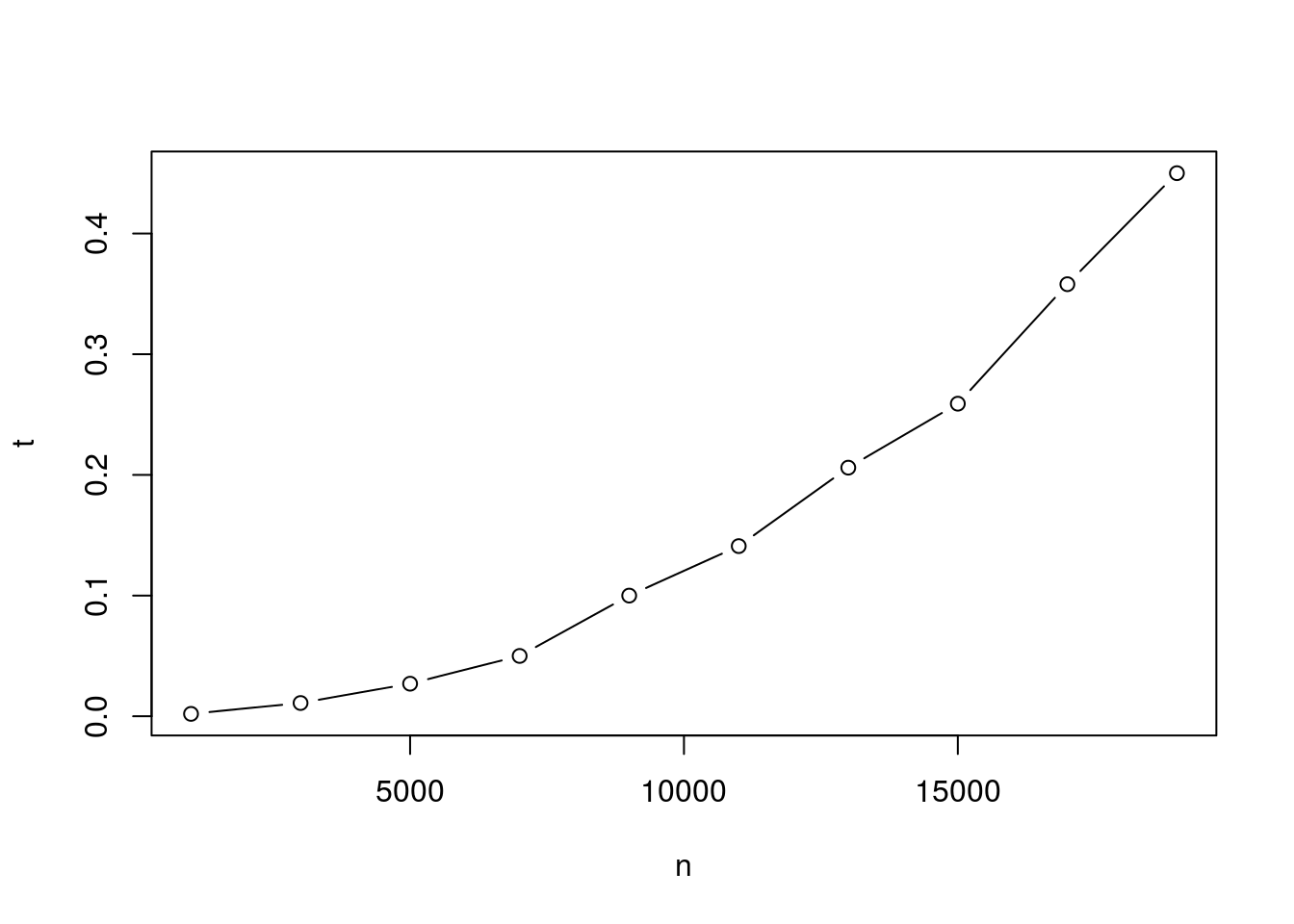Remember the current ‘correct’ value, and an approximate time

n <- 10000
system.time(expected <- f0(n))
##    user  system elapsed
##   0.121   0.000   0.120
head(expected)
##  0.000000 1.386294 2.197225 2.772589 3.218876 3.583519

Revise the function to hoist the common multiplier, a, out of the loop. Make sure the result of the ‘optimization’ and the original calculation are the same. Use the microbenchmark package to compare the two versions

f1 <- function(n, a=2) {
result <- numeric()
for (i in seq_len(n))
result[[i]] <- log(i)
a * result
}
identical(expected, f1(n))
##  TRUE
library(microbenchmark)
microbenchmark(f0(n), f1(n), times=5)
## Unit: milliseconds
##   expr      min       lq     mean   median       uq      max neval
##  f0(n) 109.7719 109.9072 130.5134 141.9948 142.5390 148.3542     5
##  f1(n) 108.5630 139.3563 133.2842 139.4147 139.4894 139.5979     5

Adopt a ‘pre-allocate and fill’ strategy

f2 <- function(n, a=2) {
result <- numeric(n)
for (i in seq_len(n))
result[[i]] <- log(i)
a * result
}
identical(expected, f2(n))
##  TRUE
microbenchmark(f0(n), f2(n), times=5)
## Unit: milliseconds
##   expr        min         lq       mean     median         uq        max
##  f0(n) 121.201141 121.739866 134.761569 143.617813 143.620317 143.628707
##  f2(n)   7.684828   7.849474   8.559228   8.415322   8.888803   9.957714
##  neval
##      5
##      5

Use an *apply() function to avoid having to explicitly pre-allocate, and make opportunities for vectorization more apparent.

f3 <- function(n, a=2)
a * sapply(seq_len(n), log)

identical(expected, f3(n))
##  TRUE
microbenchmark(f0(n), f2(n), f3(n), times=10)
## Unit: milliseconds
##   expr        min         lq       mean     median         uq        max
##  f0(n) 112.120796 143.855378 142.577849 145.567746 146.700210 178.674194
##  f2(n)   7.655573   7.698159   8.250884   8.177678   8.452503   9.566792
##  f3(n)   3.579709   3.677523   4.083759   4.048497   4.455364   4.736191
##  neval
##     10
##     10
##     10

Now that the code is presented in a single line, it is apparent that it could be easily vectorized. Seize the opportunity to vectorize it:

f4 <- function(n, a=2)
a * log(seq_len(n))
identical(expected, f4(n))
##  TRUE
microbenchmark(f0(n), f3(n), f4(n), times=10)
## Unit: microseconds
##   expr        min         lq        mean      median         uq        max
##  f0(n) 112256.477 145432.576 149337.9525 146027.0340 169576.336 179843.968
##  f3(n)   3583.222   3925.399   4090.4899   3987.5965   4017.998   5427.407
##  f4(n)    364.700    378.439    395.9539    402.8765    407.481    422.962
##  neval
##     10
##     10
##     10

Returning to our explicit iteration f2(), in these situations it can be helpful to compile the code to a more efficient representation. Do this using the compiler package.

library(compiler)
f2c <- cmpfun(f2)
n <- 10000
identical(f2(n), f2c(n))
##  TRUE
microbenchmark(f2(n), f2c(n), times=10)
## Unit: milliseconds
##    expr      min       lq     mean   median       uq     max neval
##   f2(n) 7.957343 8.582533 8.721356 8.747018 9.005959 9.45067    10
##  f2c(n) 2.000680 2.045304 2.157064 2.164375 2.216226 2.38820    10

f4() definitely seems to be the winner. How does it scale with n? (Repeat several times)

n <- 10 ^ (5:8)                         # 100x larger than f0
t <- sapply(n, function(i) system.time(f4(i))[])
plot(t ~ n, log="xy", type="b")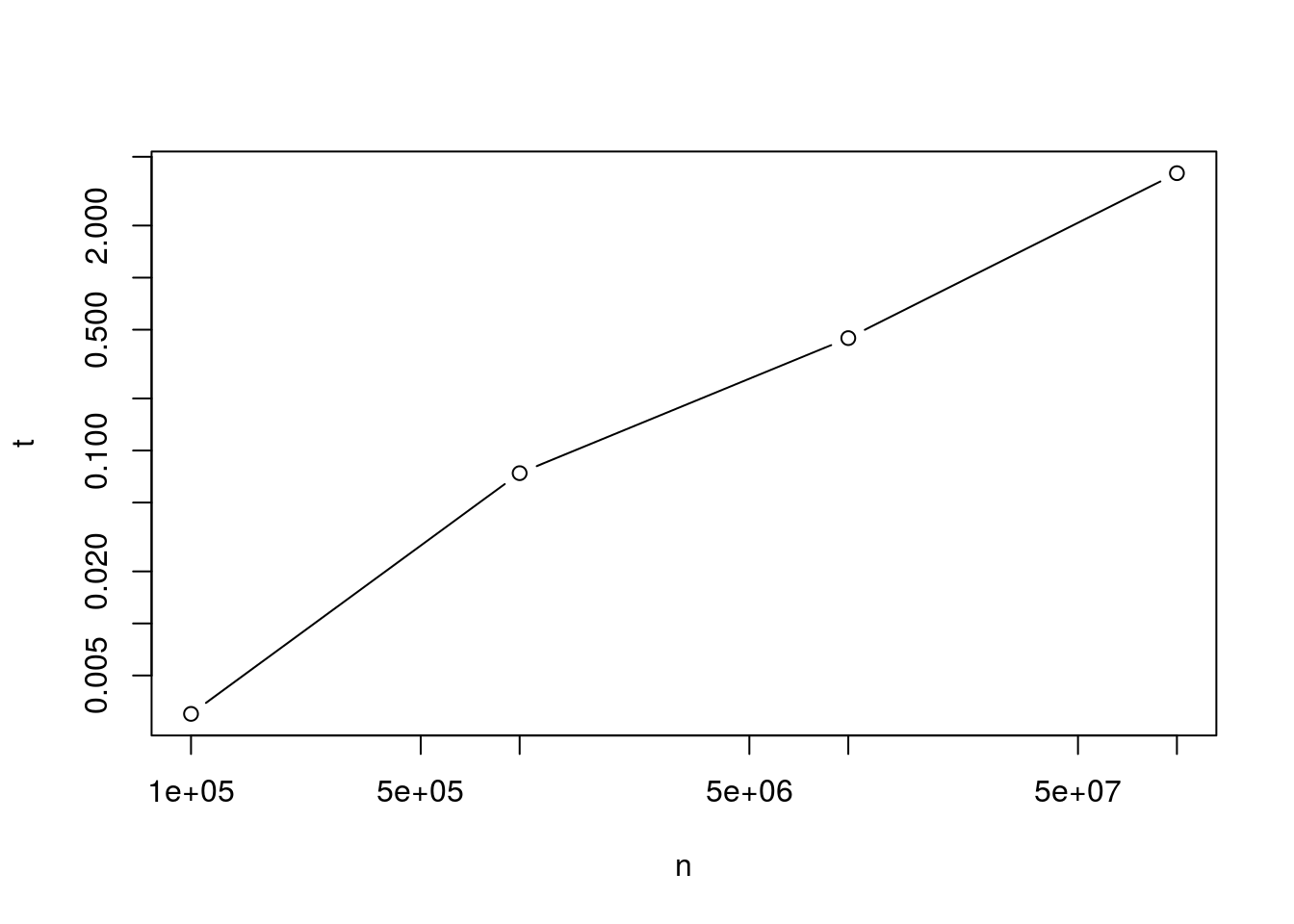Any explanations for the different pattern of response?

Lessons learned:

1. Vectorizing offers a huge improvement over iteration
2. Pre-allocate-and-fill is very helpful when explicit iteration is required.
3. *apply() functions help avoid need for explicit pre-allocation and make opportunities for vectorization more apparent. This may come at a small performance cost, but is generally worth it
4. Hoisting common sub-expressions and using the compiler package can be helpful for improving performance when explicit iteration is required.

## Case study: choosing algorithms

It can be very helpful to reason about an algorithm in an abstract sense, to gain understanding about how an operation might scale. Here’s an interesting problem, taken from StackOverflow: Suppose one has a very long sorted vector

vec <- c(seq(-100,-1,length.out=1e6), rep(0,20), seq(1,100,length.out=1e6))

with the simple goal being to identify the number of values less than zero. The original post and many responses suggested a variation of scanning the vector for values less than zero, then summing

f0 <- function(v) sum(v < 0)

This algorithm compares each element of vec to zero, creating a logical vector as long as the original, length(v). This logical vector is then scanned by sum() to count the number of elements satisfying the condition.

Questions:

1. How many vectors of length v need to be allocated for this algorithm?
2. Based on the number of comparisons that need to be performed, how would you expect this algorithm to scale with the length of v? Verify this with a simple figure.

N <- seq(1, 11, 2) * 1e6
Time <- sapply(N, function(n) {
v <- sort(rnorm(n))
system.time(f0(v))[]
})
plot(Time ~ N, type="b")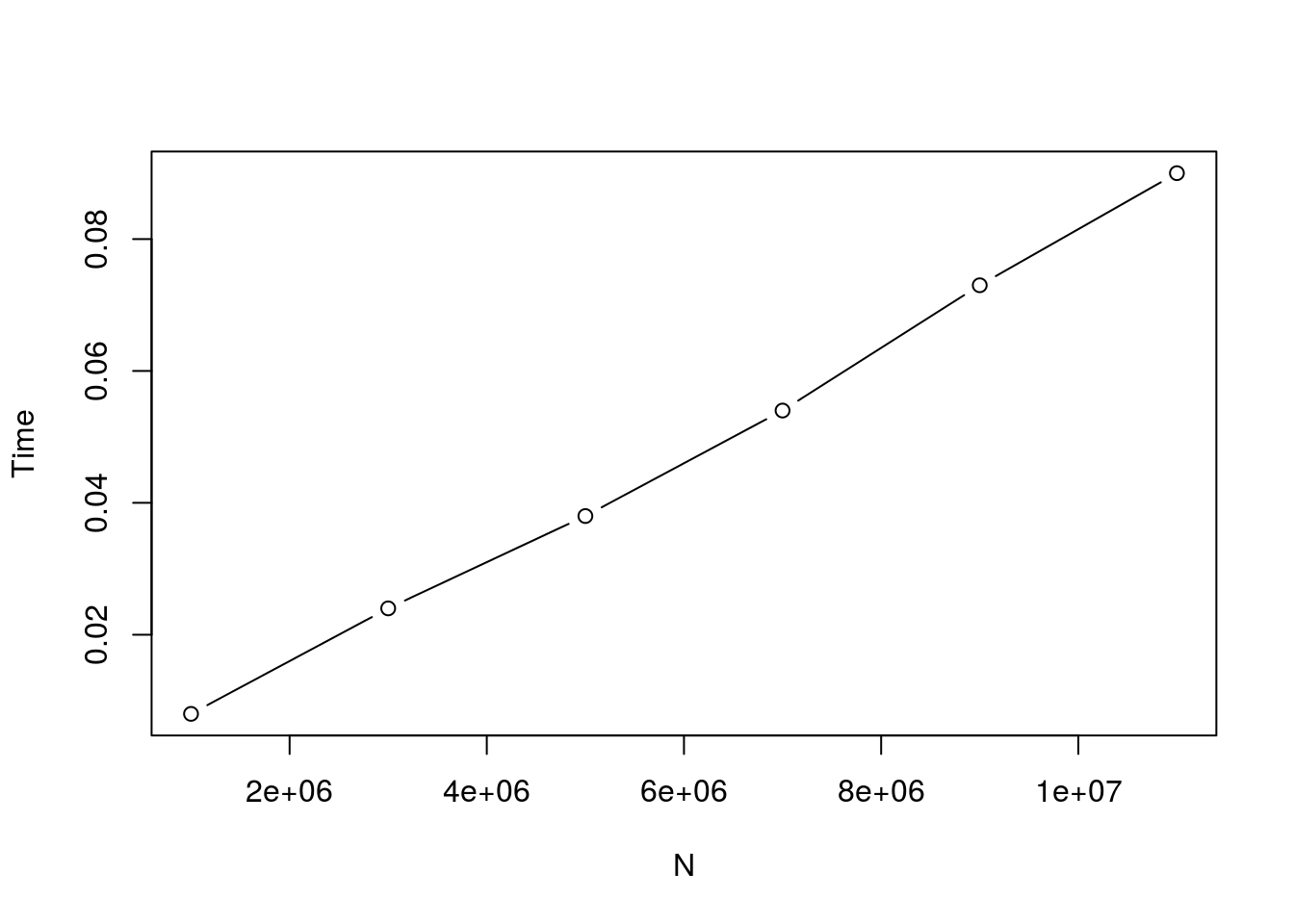Is there a better algorithm, i.e., an approach that arrives at the same answer but takes less time (and / or space)? The vector is sorted, and we can take advantage of that by doing a binary search. The algorithm is surprisingly simple: create an index to the minimum (first) element, and the maximum (last) element. Check to see if the element half way between is greater than or equal to zero. If so, move the maximum index to that point. Otherwise, make that point the new minimum. Repeat this procedure until the minimum index is no longer less than the maximum index.

f3 <- function(x, threshold=0) {
imin <- 1L
imax <- length(x)
while (imax >= imin) {
imid <- as.integer(imin + (imax - imin) / 2)
if (x[imid] >= threshold)
imax <- imid - 1L
else
imin <- imid + 1L
}
imax
}

Approximately half of the possible values are discarded each iteration, so we expect on average to arrive at the end after about log2(length(v)) iterations – the algorithm scales with the log of the length of v, rather than with the length of v, and no long vectors are created. These difference become increasingly important as the length of v becomes long.

Questions:

1. Verify with simple data that f3() and f0() result in identical() answers.
2. Compare how timing of f3() scales with vector length.

## identity
stopifnot(
identical(f0((-2):2), f3((-2):2)),
identical(f0(2:4), f3(2:4)),
identical(f0(-(4:2)), f3(-(4:2))),
identical(f0(vec), f3(vec)))

## scale
N <- 10^(1:7)

Time <- sapply(N, function(n) {
v <- sort(rnorm(n))
system.time(f3(v))[]
})
plot(Time ~ N, type="b")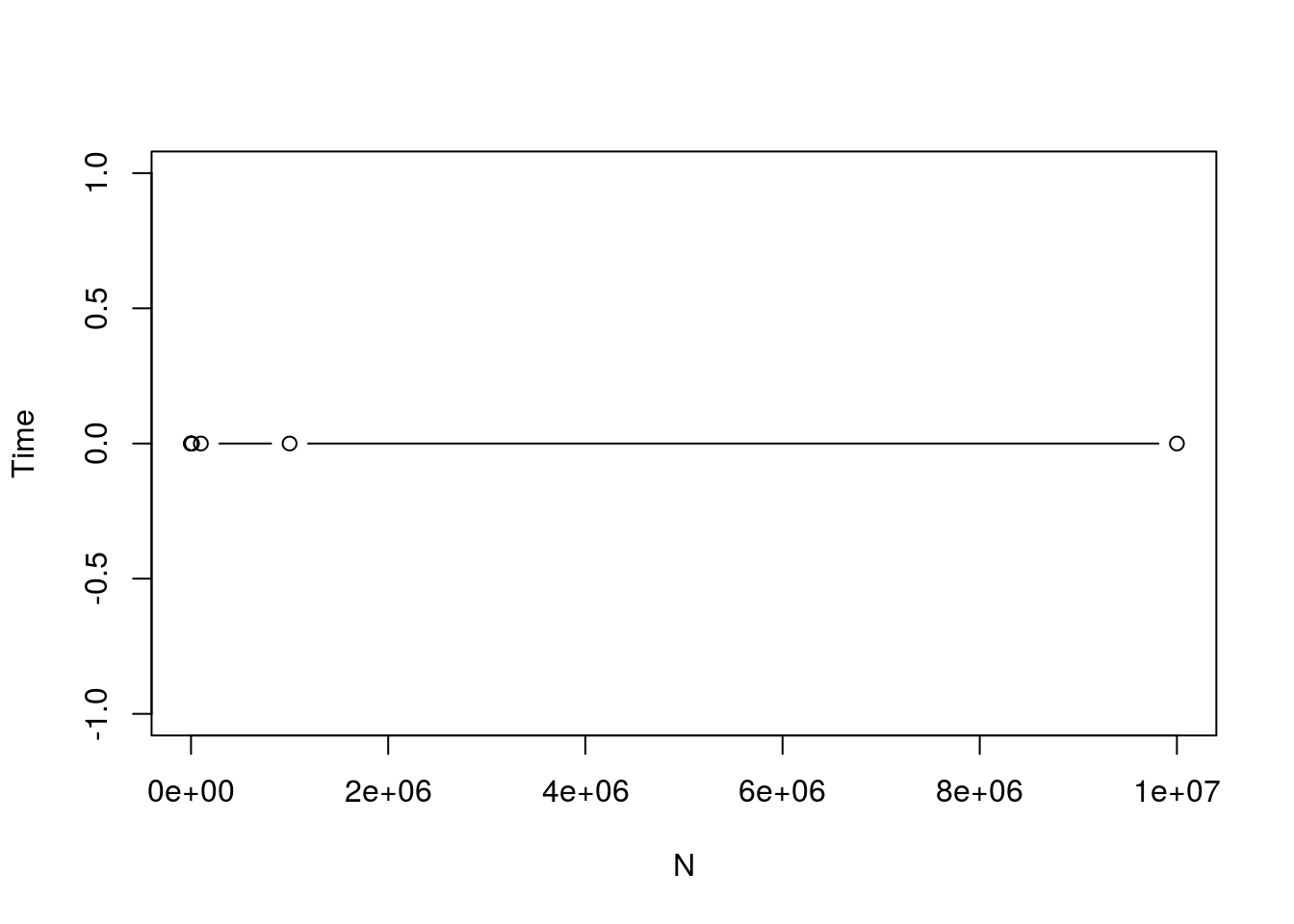3. Use the microbenchmark package to compare performance of f0() and f3() with the original data, vec.
4. R code can be compiled, and compilation helps most when doing non-vectorized operations like those in f3(). Use compiler::cmpfun() to compile f3(), and compare the result using microbenchmark.

## relative time
library(microbenchmark)
microbenchmark(f0(vec), f3(vec))
## Unit: microseconds
##     expr      min         lq        mean    median        uq       max
##  f0(vec) 15659.97 16503.9970 17429.61386 17468.603 17947.157 23199.554
##  f3(vec)    28.08    30.9575    47.23053    47.764    52.141   113.544
##  neval
##    100
##    100
library(compiler)
f3c <- cmpfun(f3)
microbenchmark(f3(vec), f3c(vec))
## Unit: microseconds
##      expr    min      lq     mean  median      uq    max neval
##   f3(vec) 28.470 29.8355 33.27442 34.6335 36.2500 48.503   100
##  f3c(vec)  6.578  7.0270  7.85183  7.3085  7.6945 18.156   100

We could likely gain additional speed by writing the binary search algorithm in C, but we are already so happy with the performance improvement that we won’t do that!

It is useful to ask what is lost by f3() compared to f0(). For instance, does the algorithm work on character vectors? What about when the vector contains NA values? How are ties at 0 treated?

findInterval() is probably a better built-in way to solve the original problem, and generalizes to additional situations. The idea is to take the query that we are interested in, 0, and find the interval specified by vec in which it occurs.

f4 <- function(v, query=0)
findInterval(query - .Machinedouble.eps, v) identical(f0(vec), f4(vec)) ##  TRUE microbenchmark(f0(vec), f3(vec), f4(vec)) ## Unit: microseconds ## expr min lq mean median uq max ## f0(vec) 15408.806 16331.254 16768.227 16472.9370 16934.660 19656.259 ## f3(vec) 28.265 30.392 44.537 43.5655 48.658 97.324 ## f4(vec) 13645.076 13698.410 13946.125 13777.6360 14172.281 15026.055 ## neval ## 100 ## 100 ## 100 The fact that it is flexible and well tested means that it would often be preferred to f3(), even though it is less speedy. For instance, compare the time it takes to query 10000 different points using f3 and iteration, versus findInterval and vectorization. threshold <- rnorm(10000) identical(sapply(threshold, f3, x=vec), f4(vec, threshold)) ##  TRUE microbenchmark(sapply(x, f3), f4(vec, x)) ## Unit: microseconds ## expr min lq mean median uq ## sapply(x, f3) 38.121 40.8785 76.45288 83.7155 89.028 ## f4(vec, x) 13650.604 13695.7095 13811.30469 13728.5565 13830.765 ## max neval ## 154.216 100 ## 14673.133 100 Some R functions that implement efficient algorithms are sort() (including radix sort), match() (hash table look-up), and tabulate(); these can be useful in your own code. Lessons learned: 1. Choice of algorithm can be very important 2. Implementing classical algorithms (like binary search) can be a rewarding learning experience even when, at the end of the day, it may be better to use existing functions. 3. The built-in R functions that implement efficient algorithms can be important building-blocks for more complicated code. # Parallel evaluation ## Case Study: GC Content of Aligned Reads This is an advanced exercise, proceed with enthusiastic caution This extended example illustrates how one might calculate the distirbution of GC content of aligned reads across several BAM files. We start by processing one BAM file sequentially, and then processes many BAM files in parallel. Find paths to the following sample BAM files (these are small, but large enough to illustrate the principle. library(RNAseqData.HNRNPC.bam.chr14) fls <- RNAseqData.HNRNPC.bam.chr14_BAMFILES ### Restriction and iteration to manage memory BAM files are large, so cannot fit into memory. In addition, we will eventually process several BAM files in parallel, so we need to further manage the amount of memory we consume while processing each BAM file. We take to approaches. The first is to iterate through the BAM file in chunks that are large enough to benefit from ’s effiecient vectorized calculation but not so large as to consume excessive memory. We do this by using BamFileList() to indicate that we would like to input aligned reads in chunks of size 100,000 library(Rsamtools) bfls <- BamFileList(fls, yieldSize=100000) Each time we read from a BAM file, we’ll input the next 100,000 records. We’ll adopt our second strategy for managing memory by restricting the data read from the BAM file to that necessary to calculate GC content, specifically the DNA sequence of each read, in addition to its alignment coordinates. We’ll do this by writing a function yield() that uses GenomicFiles::readGAlignments() to input the required data; see the help pages for functions that we use but you do not understand, e.g., ?ScanBamParam(). library(GenomicAlignments) yield <- function(bfl) { ## input a chunk of alignments library(GenomicAlignments) readGAlignments(bfl, param=ScanBamParam(what="seq")) } Next we’ll transform our aligned reads to GC content. We will do this using Biostrings::letterFrequency() to count the fraction of G’s or C’s in each read, tabulate these into 2.5-percentile bins, and calculate the cummulative number of reads in each bin. library(Biostrings) map <- function(aln) { # GC content, bin & cummulate gc <- letterFrequency(mcols(aln)seq, "GC")
tabulate(1 + gc, 73)                # max. read length: 72
}

map() will be applied to the result of each of data returned by yield(); we’ll write a function reduce() that combines the result of two calls to map() into a single summary. In our case, reduce is simply the adition of the return value of two successive calls to map().

reduce <- +

The GenomicFiles package provides a way to stitch these pieces together, specifically the reduceByYield() function, illustrated in the following code chunk

library(GenomicFiles)
bf <- BamFile(fls, yieldSize=100000)
reduceByYield(bf, yield, map, reduce)
##       0     0     0     0     0     0     0     0     0     0     0
##      0     1     1     4    24    41    87   238   490   907  1397
##   2127  3208  4706  6220  8737 11052 14680 17020 19360 21804 27047
##  29212 31249 35395 39807 40259 41722 42304 44108 44073 42317 41260
##  38372 35689 32447 27815 22153 18960 14188 10768  7887  6182  4817
##   3332  2101  1652  1455   860   459   235   116    73    34    22
##      6     4     0     0     0     0     0

The result printed out above is the number aligned reads with 0, 1, …, 73 G or C nucleotides. There are never more than 100,000 BAM records in memory at any one time, so memory consumption is modest. Nonetheless, we have processed the entire file.

### Parallel evaluation

Now that we can iterate through a single file to generate GC content in a modest amount of memory, it is very easy to process all files in parallel: use bplapply() to invoke reduceByYield() on each file, passing additional arguments yield, map, and reduce.

library(BiocParallel)
gc <- bplapply(bfls, reduceByYield, yield, map, reduce)

The result is a list of GC-count vectors, one element for each file.

### Visualization

The result can be transformed to a data.frame()

library(ggplot2)
df <- stack(as.data.frame(lapply(gc, cumsum)))
df\$GC <- 0:72

and visualized, e.g.,

library(ggplot2)
ggplot(df, aes(x=GC, y=values)) + geom_line(aes(colour=ind)) +
xlab("Number of GC Nucleotides per Read") +
ylab("Number of Reads")